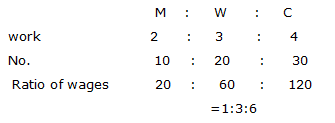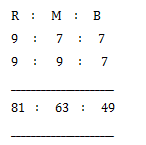# Ratio and Proportion Questions Set 11

Q.1) In a mixture of milk and water, their ratio is 5:6 respectively in the first container. And the same mixture has ratio 7:2 respectively in the second container. What is the ratio should the mixture be extracted from each container and poured in to the third container, so that the ratio of milk and water comes to 6:5 respectively in the third container?

a) 99:253

b) 253:99

c) 243:88

d) 88:243

e) None of these

b

x litres of mixture be taken from the first container and poured into the third container.

Let y litres of mixture be taken from the second container and poured into the third container.

Quantity of milk in x litres=5/11 x

Quantity of milk in y litres=7/9 y

Quantity of milk in third container = 5/11 x+7/9 y

Similarly, quantity of water in third container,

=6/11 x+2/9 y

(5x/11+7y/9)  :(6x/11+2y/9)=6 :5

((45x+77y)/99)/((54x+22y)/99)=6/5

=>324x-225x=385y-132y    =>99x=253y   =>x/y=253/99

=253 :99

Q.2) The ratio of the price of two houses was 17:24. Two years later, when the price of the first had risen by 20% while the price of second house increases by Rs.500 and their prices become 16:25. Find the original prices of the two houses together?

a) Rs.2603

b) Rs.2503

c) Rs.2403

d) Rs.2303

e) None of these

a

Let, new price of the first house=17x×120/100=102x/5

Let, new price of the second house=24x+500

According to the question,

(102x/5)  :(24x+500)=16 :25

((102x/5))/((24x+500 ) )=16/25      =>102x×5=(24x+500)16    =>x=8000/126=4000/63

Original price of first house=17×4000/63=1079.37~ Rs.1079

Original price of second house=24×4000/63=1523.81 ~ Rs.1524

Q.3) Brother A and B had some savings in the ratio 5:6. They decided to buy a gift for their sister, sharing the cost in the ratio 4:5. After they bought, A is left with three-fourth of his amount, while B is left with Rs.497. Then, the value of the gift is

a) 215

b) 115

c) 315

d) 415

e) None of these

c

Let the savings of A and B are 5x, 6x and the share cost of gift are 4y, 5y respectively.

According to question,

ForA,  5x-4y=3/4×5x     =>x=16y/5

For B,  6x-5y=497         =>6×16y/5-5y=497    =>y=35

Q.4) Nandita scores 60% marks in five subjects together, viz., Hindi, Science, Mathematics, English and Sanskrit, where in the maximum marks of each subject were 105. How many marks did Nandita score in Science, if she scored 69 marks in Hindi, 62 marks in Sanskrit, 68 marks in Mathematics and 51 marks in English?

a) 66

b) 68

c) 55

d) 65

e) None of these

d

Total of maximum marks of all subjects=105×5=525

75% of 525=525 ×60/100= 315

Obtained marks of foru subjects (Hindi, Sanskrit, mathematics and English)

=69+62+68+51=250

So, the obtained marks in Science=315-250=65

Ans=d) 65

Q.5) Mr. Shrimant inherits 4325 gold coins and divides them among his three sons; Bharat, Parat and Marat; in a certain ratio. Out of the total coins received by each of them, Bharat sells 40 coins; Parat donates his 20 coins and Marat loses 30 coins. Now, the ratio of gold coins with them is 41:34:46, respectively. How many coins did Parat receive from his father?

a) 1210

b) 1211

c) 1212

d) 1213

e) None of these

a

According to question

41x+40+34x+20+46x+30=4325      =>121x=4325-90     =>x=35

number of coins recevied by parat

=34x+20=34×35+20=1210

Q.6) The sum of the ages of the 4 members of Sinha family is 172 years. 8 years ago the ages of the 4 members Nishu, Vicky, Mrs.Sinha and Sinha were in the ratio of 2:3:7:8. After how many years would Nishu be as old as the present age of his mother?

a) 33 years

b) 35 years

c) 36 years

d) 37 years

e) None of these

b

Let their ages 8 years ago be 2x, 3x, 7x, and 8x.

Their ages now 2x+8, 3x+8, 7x+8, 8x+8.

According to the question,

=2x+8+3x+8+7x+8+8x+8=172

20x+32=172    =>x=140/20=7

Present age of Nishu=2×7+8=22 years

Present age of mother=7×7+8=57 years

Hence, required years (57-22)=35 years

Q.7) The work done by a man, a woman and a child is in the ratio of 2:3:4. There are 10 men, 20 women and 30 children in a factory. Their weekly wages amount to Rs.1600, which is divided in the ratio of work done by the men, women and children. What will be the wages of 15 men, 21women, and 30 children for 2 weeks?

a) 2270

b) 2440

c) 3408

d) 3230

e) None of these

cTotal Wages of a men=1/10×1600=160

Wages of a men=160/10  =16

Similarly, women→24;     Child→32;

Total wages of 15 men, 21 women and 30 children=15×16+21×24+30×32=240+504+960=1704

Total wages of 2 weeks=1704×2=3408

Q.8) A bus and a truck are available to cross a jungle. The speed of the truck is thrice that of the bus. The capacity of the truck is 60 persons and that of bus is 40 persons. The average occupancy of the bus is twice that of the truck. The tickets for the bus and the truck cost Re 1 and Re 1.50 respectively. What is the ratio of the average rupee collection of the truck to that of the bus in a day? Assume there is no wastage time between trips and the occupancy of the bus/truck is defined as the ratio of the actual number of persons boarding it and its capacity.

a) 9:17

b) 17:9

c) 8:27

d) 27:8

e) None of these

d

Average Rupee collection = Speed× capacity × Occupancy × Ticket rate
ratio of average Rupee collection of truck to that of bus= product of above rate

According to question,

(3×60×1×1.5):(1×40×2×1)=  270:80=27:8

Ans=d) 27:8

Q.9) Mani, Ram and Bhuvana have some stones with each of them. Seven times the number of stones with Ram equals nine times the number of stones with Mani while seven times the number of stones with Mani equals nine times the number of stones with Bhuvana. What is the minimum number of stones that can be there with all three of them put together?

a)193

b) 195

c) 197

d) 199

e) None of these

a

Let the stones with Mani, Ram and Bhuvana be M, R and B respectively.
Given, 7R=9M;    7M=9B

R/M=9/7;   M/B=9/7   =>so, R:M=9:7;     M:B=9:7The least possible integral values for R, M, B will be R=81, M=63 and B=49

=>Total=81+63+49=193

Q.10) A, B and C invest in a business. If the ratio of their time periods are 4 : 5 : 6 and their profits are in the ratio 7 : 8 : 9. Find the ratio in which the investment are made by A, B and C.

a) 45 : 35:27

b) 35 : 32 : 30

c) 27 : 37 : 44

d) 30 : 32 : 35

e) 41 : 42 : 34

b

Ratio of their investment

=7/4  : 8/5  : 9/6     =>take LCM

=7×15 :8×12 :9×10

A :B :C= 35 :32 :30